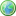Accessibility options:

# Differential Equations resources

Show me all resources applicable to

### Quick Reference (2)Solving Differential Equations by Separating Variables
Quick Reference leaflet on first order differential equations. This Quick Reference leaflet is contributed to the mathcentre Community Project by Katy Dobson and reviewed by Alan Slomson, University of Leeds.Solving Differential Equations with Integrating Factors
Quick Reference leaflet on solving differential equations. This Quick Reference leaflet is contributed to the mathcentre Community Project by Katy Dobson and reviewed by Alan Slomson, University of Leeds.

### Teach Yourself (2)First Order Ordinary Differential Equations
First Order Differential Equations: A summary of five common methods to solve first order ODEs: direct integration, separation of variables, homogeneous equations, integrating factor and Bernouilli equations. This Teach Yourself resource is contributed to the mathcentre Community Project by Morgiane Richard, University of Aberdeen and is reviewed by Shazia Ahmed, University of Glasgow.Second Order Ordinary Differential Equations
Second Order Differential Equations: A summary of how to solve second order ODEs with constant coefficients: how to solve homogeneous equations (with no right hand-side) and how to solve equations with a right hand-side (method of undetermined coefficients and methods of variation of parameters). This Teach Yourself resource is contributed to the mathcentre Community Project by Morgiane Richard, University of Aberdeen and is reviewed by Shazia Ahmed, University of Glasgow.

### Test Yourself (4)Differential Equations Test 01 (DEWIS)
Four questions on second order linear constant coefficient differential equations. The first two involve identifying the complementary function, the third involves applying initial conditions and the fourth involves finding a particular solution with either linear or sinusoidal forcing. DEWIS resources have been made available under a Creative Commons licence by Rhys Gwynllyw & Karen Henderson, University of the West of England, Bristol.First order differential equations - Numbas
9 questions on first order differential equations. Straight forward integration (2), separating variables (4), linear (1), homogenous (2). All are either initial value or boundary value problems. Numbas resources have been made available under a Creative Commons licence by Bill Foster and Christian Perfect, School of Mathematics & Statistics at Newcastle University.First order differential equations - Numbas
9 questions on first order differential equations. Straight forward integration (2), separating variables (4), linear (1), homogenous (2). All are either initial value or boundary value problems. Numbas resources have been made available under a Creative Commons licence by the School of Mathematics & Statistics at Newcastle University.Second order differential equations - Numbas
5 questions on second order differential equations. First two and last, linear with constant coefficients; first two homogeneous, complex and repeated roots. Last, non-homogeneous. Third, motion under gravity. Fourth, linear with a given particular solution (variation of parameters). Numbas resources have been made available under a Creative Commons licence by the School of Mathematics & Statistics at Newcastle University.

Website design by Pink Mayhem, Leicester